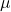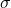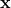In this blog, we will walk through a probabilistic formulation of the well-known technique of principal component analysis (PCA). The probabilistic PCA (PPCA) was introduced by Bishop . PPCA does not generate better results than PCA, but it permits a broad range of future extensions. Besides, PPCA falls in the category of Bayesian models, which are enjoying a renaissance. Other models, such as Gaussian mixture model, K-means clustering, factor analysis, and variational autoencoder, are all Bayesian models or can be viewed from a Bayesian perspective. So I hope this blog helps to view PCA from a Bayesian angle and grasp the basic framework of constructing a Bayesian model. In the following parts, I will briefly introduce PCA, then focus on how PPCA was formulated, how to train PPCA and inference in PPCA.

PCA is a dimensionality reduction technique that transforms the original data into a lower dimensionality space via an orthogonal transformation. PCA can be derived in two ways. One is to find a manifold of lower dimensionality that the data have a maximum variance when the data is projected in the lower dimensionality space. The idea of the other way is to minimize recovery error if we try to recover the original data from the compressed data.

PPCA is formulated as a Bayesian generative model. In such a framework, we define an explicit latent variable z corresponding to the principal-component subspace. Next, we relate the latent variable to the observed variable, x, via Bayesian theorem, which defines a rule to update the posterior distribution using conditional distribution and prior. We choose Gaussian distribution as the prior and conditional distribution due to the desired properties of Gaussian distribution. The PPCA is fully defined by the following six equations in which a lowercase/uppercase letter in bold represents a vector/matrix. First, note that equation 13 are assumptions and equation 46 are derived using the properties of Gaussian distribution. Here I describe the essence of each equation in one sentence.

1. We assume the latent variable zis given by a zero-mean unit-covariance Gaussian.

2. We generate a higher-dimensional x via a mapping matrix W, plus a vectorand some noise.

3. Equation 3 defines a Gaussian variable, which is the noise part in equation 2.

4. The distribution of x, conditional on the latent variable z, follows a Gaussian distribution, whose mean and covriance matrix areand, respectively.,, andare parameters that will be learned during the training process.

5. The integral of the joint distribution ofandoveryields the distribution of, which follows a Gaussian distribution, whose mean () and covariance matrix () are equal to,and, respectively. If you don’t care about the math details, the takeaway from this equation is thatfollows a Gaussian.

6. Equation 2 defines a way to mapto. To “map”to, in a probabilistic language, we need to the distribution ofconditional on,. Due to properties of Gaussian distribution,also follows a Gaussian distribution, whose mean is equal to. Theis not used in inference, so I omit it here.,,are the same as before.

(1)(2)(3)(4)(5)(6)So far, we have defined PPCA. Now it is time to train PPCA. Since the marginal likelihood ofin equation 4 has an analytic form. We can maximize thedirectly. An alternative way is to use the expectation-maximization (EM) algorithm which sequentially optimizeswith respect to the distribution of z and with respect to the parameters in each iteration. (EM is commonly used when training a latent variable model.)

Finally, with the trained parameters,,,, we can use equation 6 to map \mathbf{x} to a lower-dimension space. For further details about the derivation and implementation in Python, see the references.

References:
1. Bishop, Christopher M. Pattern recognition and machine learning. springer, 2006.2. https://www.cs.toronto.edu/~rsalakhu/STA4273_2015/notes/Lecture8_2015.pdf
3. https://github.com/AndriiDSD/PPCA